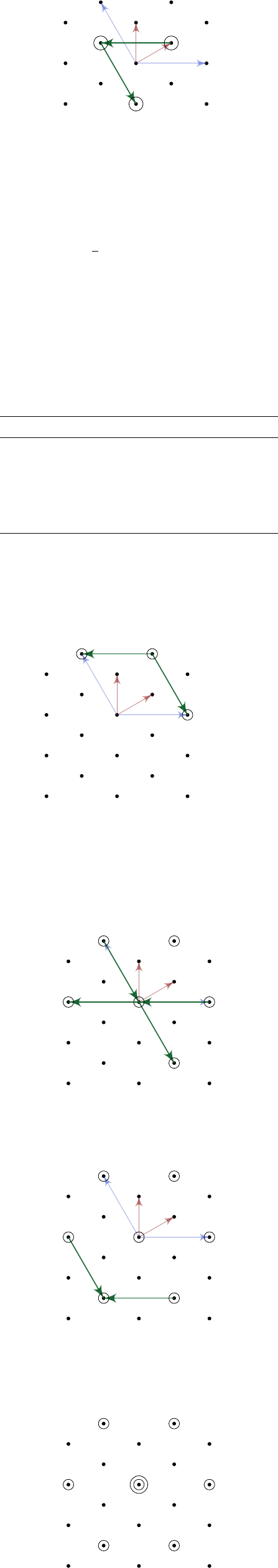6Representation of Lie algebras

III Symmetries, Fields and Particles6.3 Classification of representations
Suppose we have a representation
ρ
of
g
v V
λ
. We then keep applying things of the form
ρ
(
E
α
), where
α
Φ
+
is a
positive weight. Doing so will bring us further away from the hyperplane dividing
the positive and negative weights, and since there are only finitely many weights,
we must eventually stop. This is known as a highest weight.
Definition
(Highest weight)
.
A highest weight of a representation
ρ
is a weight
Λ S
ρ
whose associated eigenvector v
Λ
is such that
ρ(E
α
)v
Λ
= 0
for all α Φ
+
.
The Dynkin labels (or indices) of a representation are the Dynkin labels of
its highest weight.
Now if v V
λ
, then we know
ρ(E
α
)v V
λ+α
,
if
λ
+
α S
ρ
(and vanishes otherwise). So acting with the step operators
translates us in the weight lattice by the vector corresponding to the root.
In the case of
su
(2), we found out an explicit formula for when this will stop.
In this general case, we have the following result which we will not prove:
Theorem. For any finite-dimensional representation of g, if
λ =
r
X
i=1
λ
i
ω
(i)
S
ρ
,
then we know
λ m
(i)
α
(i)
S
ρ
,
for all m
(i)
Z and 0 m
(i)
λ
i
.
If we know further that
ρ
is irreducible, then we can in fact obtain all weights
by starting at the highest weight and applying this procedure.
Moreover, for any
Λ =
X
Λ
i
ω
(i)
L
W
[g],
this is the highest weight of some irreducible representation if and only if Λ
i
0
for all i.
This gives us a very concrete way of finding, at least the weights, of an
irreducible representation. In general, though, we don’t immediately know the
multiplicities of the weights.
Definition
(Dominant integral weight)
.
A dominant integral weight is a weight
Λ =
X
Λ
i
ω
(i)
L
W
[g],
such that Λ
i
0 for all i.
Example.
Take the example of
g
=
A
2
. It is a fact that the fundamental
representation f has Dynkin labels (1, 0). In other words,
Λ = ω
(1)
.
To get the remaining weights, we subtract roots:
(i) Λ = ω
(1)
S
f
. So we can subtract by α
(1)
exactly once.
(ii) So
λ = ω
(1)
(2ω
(1)
ω
(2)
)
= ω
(1)
+ ω
(2)
S
f
.
This has Dynkin labels (
1
,
1). So we can do one further subtraction to
get a new weight
λ α
(2)
= ω
(1)
+ ω
(2)
(2ω
(2)
ω
(1)
)
= ω
(2)
In the weight diagram, these are given by
ω
(2)
ω
(1)
α
β
(1, 0)
(1, 1)
(0, 1)
In general, for a dominant integral weight of the form
Λ = Λ
1
ω
(1)
+ Λ
2
ω
(2)
L
W
[A
2
]
for Λ
1
, Λ
2
Z
0
. We then get an irrep ρ
1
,Λ
2
)
of A
2
.
One can show, via characters, that the dimension of this representation is
given by
dim ρ
1
,Λ
2
)
=
1
2
1
+ 1)(Λ
2
+ 1)(Λ
1
+ Λ
2
+ 2).
This is symmetric in Λ
1
and Λ
2
. So if Λ
1
6
= Λ
2
, then we get a pair of distinct
representations of the same dimension. It is a fact that
λ S
1
,Λ
2
)
λ S
2
,Λ
1
)
,
and we have
ρ
1
,Λ
2
)
= ¯ρ
2
,Λ
1
)
.
On the other hand, if Λ
1
= Λ
2
, then this representation is self-conjugate.
The first few representations of A
2
are given by
Highest weight Dimension Name
ρ
(0,0)
1 Trivial
ρ
(1,0)
3 Fundamental
ρ
(0,1)
¯
3 Anti-fundamental
ρ
(2,0)
6
ρ
(0,2)
¯
6
ρ
(1,1)
We can figure out the weights of the adjoint representation with Λ = (1
,
1)
S
ρ
.
Since both labels are positive, we can subtract both α
(1)
and α
(2)
to get
Λ α
(1)
= (1, 2)
Λ α
(2)
= (2, 1)
ω
(2)
ω
(1)
α
β
(1, 1)(1, 2)
(2, 1)
Now we have a +2 in the Dynkin labels. So we can subtract twice. So we have
the following weights:
Λ α
(1)
α
(2)
= (0, 0)
Λ α
(1)
2α
(2)
= (1, 2)
Λ 2α
(1)
α
(2)
= (2, 1)
ω
(2)
ω
(1)
α
β
(1, 1)(1, 2)
(2, 1)
(0, 0)
(1, 2)
(2, 1)
Finally, we can subtract α
(1)
from the second or α
(2)
from the third to get
Λ 2α
(1)
2α
(2)
= (1, 1) S
ρ
.
ω
(2)
ω
(1)
α
β
(1, 1)(1, 2)
(2, 1)
(0, 0)
(2, 1)
(2, 1)
(1, 1)
Now notice that we only have seven weights, not eight. This is because our
algorithm did not tell us the multiplicity of the weights. However, in this
particular case, we know, because this is the adjoint representation, and the
Cartan generators give us two things of weight 0. So we can plot all weights
with multiplicity as
It is a general fact that the weight lattice traces out a polygon like this, and
the vertices at the perimeter are always non-degenerate. So in this case, we
could also have figured out the multiplicty by using this general fact.